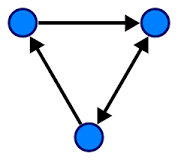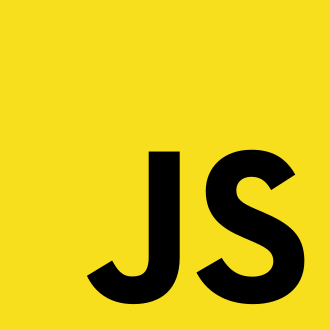# Algorithm Study #6 (Graph_Concept)

jakeseo_me·2019년 3월 12일
0

목록 보기
15/18

## Graph• it's a type of datastructure
• it has Node and Vertex(정점), Edge(간선)
- Vertex and Node mean same thing. Circled ones in the image.
- Edge means lines connecting circles.
- This represents the relationship between vertices.
• G = (V, E)
- friends are vertices
• relationships are edges

## Path in Graph• it has 5 Vertices and 7 Edges
• Path from A to B
- A->C->D->E->B
• A->B
• A->C->B
• A->C->E->B

## Cycle in Graph

• This refers to a case where the origin and destination are the same.
- A to A
• B to B
• Cycle about A to A
- A->C->B->A
• A->C->E->B->A
• A->C->D->E->B->A

## what we usaully need in Graph

• we usually need some shortest path
• express conditions as a graph and solve it

## Simple Path and Simple Cycle

• Graph which is not visiting the same vertex more than once in a path or cycle
• When nothing is mentioned, the commonly used path or cycle is this case.

## Directed Graph

• if there is arrow at the end of the edges
• in case of graph above
- A->C is possible
• but C->A is impossible

## Undirected Graph

• there is no arrow at the end of the edges
• it is also called Bidirected Graph
• when it comes to code, write about two edges

## Mutiple Edge

• there can be multiple edges between two vertices
• if there are two edges which has different weight
- smaller weight one would be win and bigger weight one would be ignored

## Loop edge

• it also can be possible that one edge's start point and end point are the same.
- A -> A

## Weight

• when there is weight, it is a really important thing.
• it is about how much it will cost or take...
• if there is no weight, we can think that all weights get value of 1

## Degree

• Degree means edges of each vertice
• if a has 3 edges, a's degree is also 3
• in case of directed graph
- it is divided into indegree and outdegree대전에 있는 (주) 아이와즈에서 풀스택 웹개발자로 일하고 있는 서진규입니다. 주로 Jake Seo라는 닉네임을 많이 씁니다.